Disclaimer: This is an example of a student written essay.

Any scientific information contained within this essay should not be treated as fact, this content is to be used for educational purposes only and may contain factual inaccuracies or be out of date.

# Solving the Redundancy Allocation Problem using Tabu Search

 ✅ Paper Type: Free Essay ✅ Subject: Engineering ✅ Wordcount: 2090 words ✅ Published: 30th Aug 2017

Efficiently Solving the Redundancy Allocation Problem using Tabu Search

Abstract

The redundancy allocation problem is a common and extensively studied program involving system design, reliability engineering and operations research. There is an ever increasing need to find efficient solutions to this reliability optimization problem because many telecommunications (and other) systems are becoming more complex while the development schedules are limited. To provide solutions to this, a tabu search meta-heuristic has been developed and successfully. Tabu search is a perfect solution to this problem as it has a lot of advantages compared to alternative methods. Tabu search can be used for more complex problem domain compared to the mathematical programming methods. Tabu search is more efficient than the population based search methodologies such as genetic algorithms. In this paper, Tabu search is used on three different problems in comparison to the integer programming and genetic algorithm solutions and the results show that tabu search has more benefits while solving these problems.

1. INTRODUCTION of Articles

Redundancy allocation problem(RAP) is a popular and a complex reliability design problem. The problem has been solved using different optimization approaches. Tabu search(TS) has more advantages over the other approaches but has not been tested for its effectiveness. In this paper a TS is used to solve a problem, called TSRAP, and the results are compared to the other approaches.

The RAP is used for designs that have large assemblies and are manufactured using off-the shelf components and also have high reliability requirements. Solutions to the RAP problem has the optimal combination of component selections. Mathematical programming techniques have proven to be successful in finding solutions to these problems. Unfortunately, these problems have some constraints which are necessary for the optimization process but not for the actual engineering design process. Genetic Algorithms have proven to be a better alternative to the mathematical programming technique and has provided excellent results. Despite this, genetic algorithms is a population based search requiring the evaluation of multiple prospective solutions because of which a more efficient approach to this problem is desired.

If you need assistance with writing your essay, our professional essay writing service is here to help!

TS is an alternative to these optimization methods that has been optimized by GA. It’s a simple solution technique that proceeds through successive iterations by considering neighboring moves. In this paper the TS method is used on three different problems and the results are compared with the alternate optimization methods. TS is not like GA, which is population based, instead it successively moves from solution to solution. This helps increase the efficiency of the method.

The most commonly studied design configuration for RAP is the series parallel problem. The example of the design is shown below.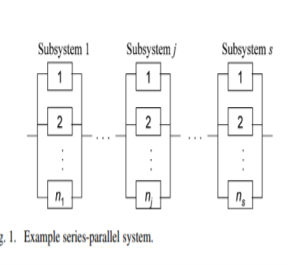Nomenclature

R(t, x) = system reliability at time t, depending on x;

xij = quantity of the jth available component used

in subsystem i;

mi = number of available components for subsystem

i;

s = number of subsystems;

nmax,i = ni â‰¤ nmax,iâˆ€i;

C(x) = system cost as a function of x;

W(x) = system weight as a function of x;

C, W, R = system-level constraint limits for cost,weight,

and reliability;

k = minimum number of operating components

required for subsystem;

Î»ij = parameter for exponential distribution,

fij(t) = Î»ij exp(âˆ’Î»ijt);

Fj = feasible solutions contained on the tabu list;

Tj = total number of solutions on the tabu list;

Ïj = feasibility ratio, Ïj = Fj/Tj .

1. Explanation of the work presented in journal articles

The RAP function can be formulated with system reliability as the objective function or in the constraint set. Problem(p1) maximizes the system reliability and problem(p2) maximizes the system cost.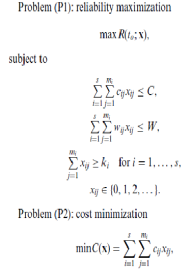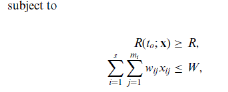The TS requires determination of a tabu list of unavailable moves as it successively proceeds from one step to another. For the series parallel system, the encoding is a permutation code of size âˆ‘i=1 s nmax, I representing the list of components in each subsystem including nonused components. The tabu list length is reset every 20 iterations to an integer value distributed uniformly between [s, 3s] and [14s,18s] for Problems (P1) (s = 14) and (P2) (s = 2), respectively.

Our academic experts are ready and waiting to assist with any writing project you may have. From simple essay plans, through to full dissertations, you can guarantee we have a service perfectly matched to your needs.

TSRAP is done through four steps. The first step involves generating a feasible random initial solution. S integers are chosen from the discrete uniform distribution, representing the number of components in parallel for each subsystem. Using this procedure, a solution is produced with an average number of components per subsystem. It becomes the initial solution if feasible, else the whole process is repeated.

The second step checks for possible defined moves for each subsystem in the neighborhood. The TSRAP that allows component mixing within the subsystem allows for its first move to change the number of a particular component type by adding or subtracting one. The TSRAP that does not allow component mixing involves changing the number of components by adding or subtracting one for all individual subsystems. These moves are advantageous as they do not require re-calculation of the entire system reliability. The best among the two types of moves that are performed independently are selected. The selected move is the best move available, hence it is called best move. If the solution is TABU and the solution is not better than the best so far solution then it is disallowed and step 1 is repeated, else it is accepted.

The third step involves updating the Tabu list. To check for the feasibility of an entry in the Tabu list, the system cost and weight are stored with the subsystem structure involved in the move within the tabu list.

The fourth and the final step is checking for the stopping criterion. It is the maximum number of iterations without finding an improvement in the best feasible so far. When reached at a solution, the search is completed and the best feasible so far is the is the TSRAP recommended solution.

An adaptive penalty method has been developed for problems solved by TS as they prove to give better solutions. The objective function for the infeasible solution is penalized by using subtractive or additive penalty function. A light penalty is imposed on the infeasible solutions within the NFT region( Near Feasible Treshold) and heavily penalized beyond it. The penalized objective function is based on the unpenalized objective function, the degree of infeasibility and information from the TS short-term and long-term memory. The objective function is for problem 1: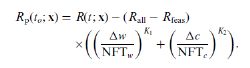Rp(to;x) is the penalized objective function. The un penalized system reliability of the best solution so far is represented by Rall and Rfeas represents the system reliability of the best feasible solution found so far. If Rall and Rfeas are equal or close to each other in value then the search continues, else if Rall is greater then Rfeas, there is a difficulty in finding the feasible solutions and the penalty is made larger to filter the search into the feasible region.

Similarly, the objective function for problem 2 is: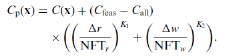Cp(x) is the penalized objective function. Call is the unpenalized (feasible or infeasible) system cost of the best

solution found so far, and Cfeas is the system cost of the

best feasible solution found so far.

1. Discussion of Contributions

The most important contribution is that as a result of this paper it is now proved that the Tabu search is a more efficient method that the mathematical programming technique and the genetic algorithms. The penalization method was used which proved to give better results too. As a result of this paper, complex problem domains can now be optimized better using the Tabu search. As a result of this paper, we’ve come to realize that TSRAP is better in performance and results in greater efficiency than GA although they are almost similar in procedures. Due to the short schedules to find the optimal solution for complex redundancy allocation problems, Tabu search is found to be the most efficient approach.

1. Discussion of Dificiency and Potential Improvements

Although an unexploited approach to find the optimal solution has been tried and tested to be efficient, there is potential for future scope. In this paper , the TS approach used is rather simple in a way that few factors that could have been were not incorporated. Features that are normally used such as candidate lists and long term memory strategies which prove to be more effective were not used. The use of these features can prove to be more efficient in complex problems. There are opportunities for improved effectiveness and efficiency by considering the addition of these features to the TS devised here.

1. Summary

TS has previously been demonstrated to be a successful

optimization approach for many diverse problem domains. So, TS approach , as a result of this paper has been tried and tested to be more efficient approach to the complex problems domain of the redundancy allocation problem. The use of penalty function in this research has promoted the search in the infeasible region by changing the NFT. In this paper, TS has been tested in three different problems and has provided more efficient results than the other alternative methods. When compared, the TS produces better results than the genetic algorithm method.

In spite of this, the use of features such as candidate lists and long term memory strategies could have been to be more effective in complex problem domains.

References

Bellman, R.E. and Dreyfus, E. (1962) Applied Dynamic Programming, Princeton University Press, Princeton, NJ.

Bland, J.A. (1998a) Memory-based technique for optimal structural design. Engineering Applications of Artificial Intelligence, 11(3), 319- 325.

Bland, J.A. (1998b) Structural design optimization with reliability constraints using tabu search. Engineering Optimization, 30(1), 55-74.

Brooks, R.R., Iyengar, S.S. and Rai, S. (1997) Minimizing cost of redundant sensor-systems with non-monotone and monotone search algorithms, in Proceedings of the Annual Reliability and Maintainability Symposium, IEEE, New York, pp. 307-313.

Bulfin, R.L. and Liu, C.Y. (1985) Optimal allocation of redundant components for large systems. IEEE Transactions on Reliability, 34, 241-247.

Chern, M.S. (1992) On the computational complexity of reliability redundancy allocation in a series system. Operations Research Letters,11, 309-315.

View all

## DMCA / Removal Request

If you are the original writer of this essay and no longer wish to have your work published on UKEssays.com then please:

Related Services

Prices from

SR571

Approximate costs for:

• 1000 words
• 7 day deliveryHumanity University

Dedicated to your worth and value as a human being!

Related Lectures